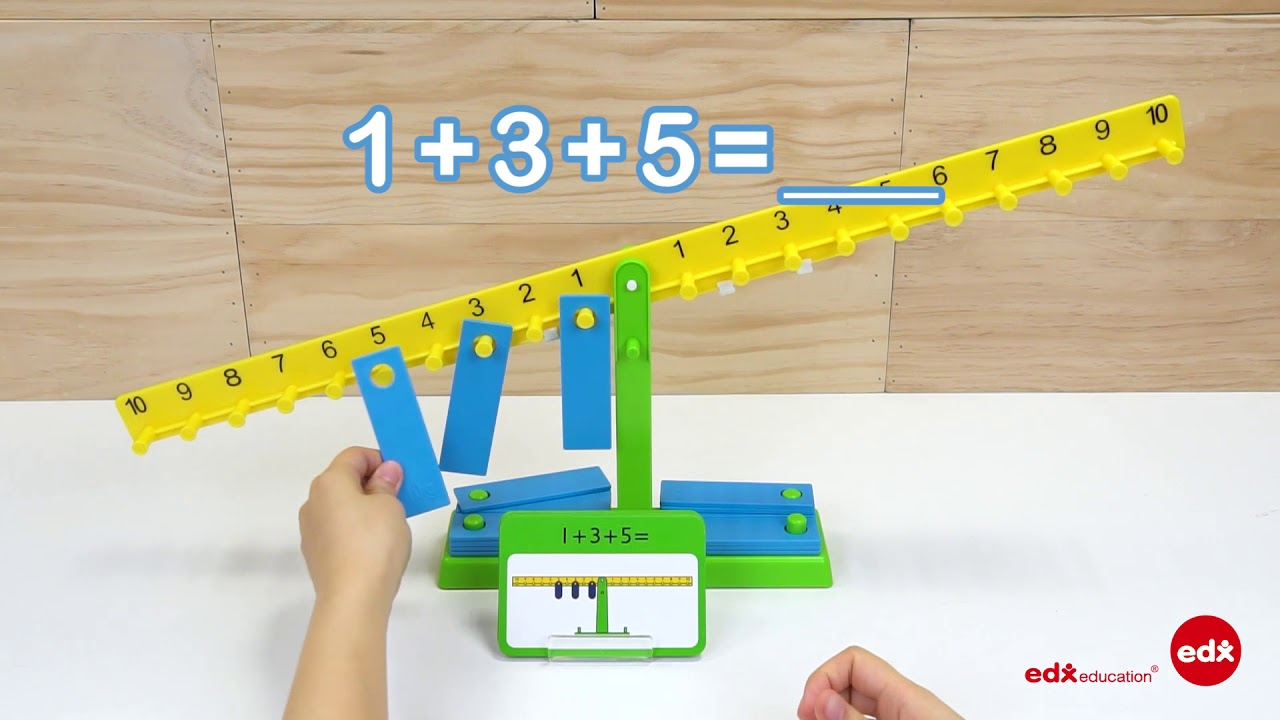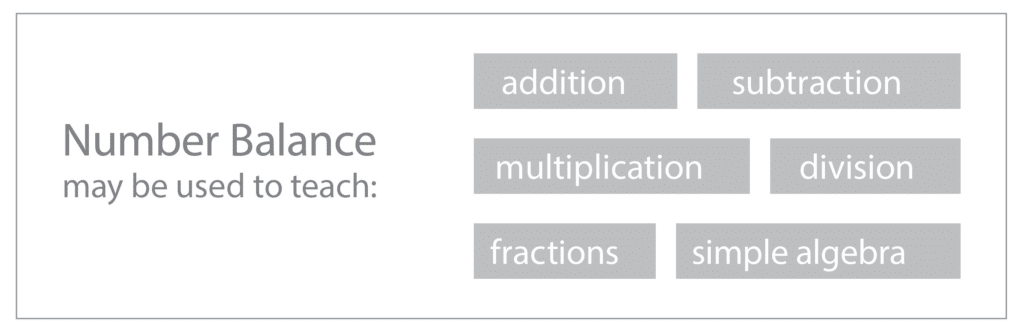# Number Balance

#### • Algebra: Equations, Equivalence

The Number Balance relies on the principle of equivalence. Weights are placed on either side of the fulcrum or pivot point. If a weight is placed on the peg marked 8 on the right arm of the balance then it may be ‘balanced’ by placing a weight on 6 and another on 2 on the left arm of the balance. The equation 8 = 6 + 2 is formed. Six may be balanced with 5 and 1, and by placing two weights on 3. This basic idea may be extended into early algebra.

### Number Balance Videos### Mathematical Language

Balance, equivalent, fulcrum, pivot, weighs more, weighs less, weighs the same.

### Using the Number Balance

The Number Balance may be used to learn about addition and particularly about the commutative property of addition, that is 6 = 4 + 2 and 2 + 4 = 6. Links to equations such as 2 + 2 + 1 + 1 = 4 + 2 may be made. The ideas of greater than and less than may be explained using a number balance. subtraction, multiplication and division may all be explored using a number balance. Later early algebra concepts may be introduced.Greater than / less than

Subtraction

Multiplication

Division